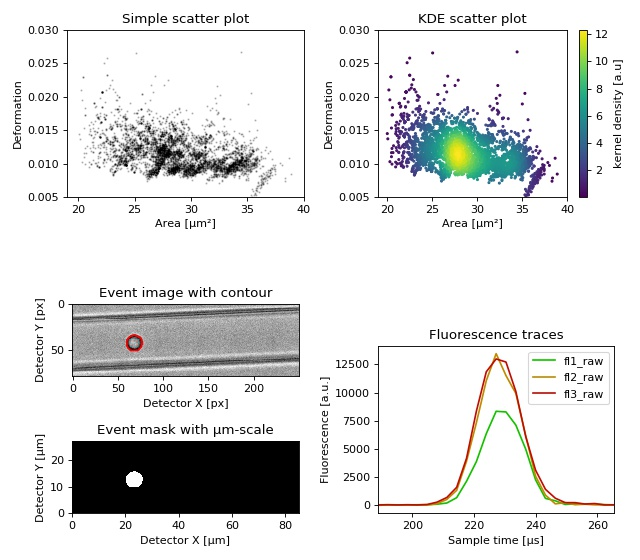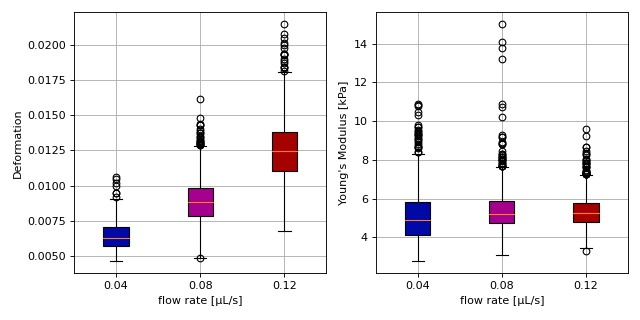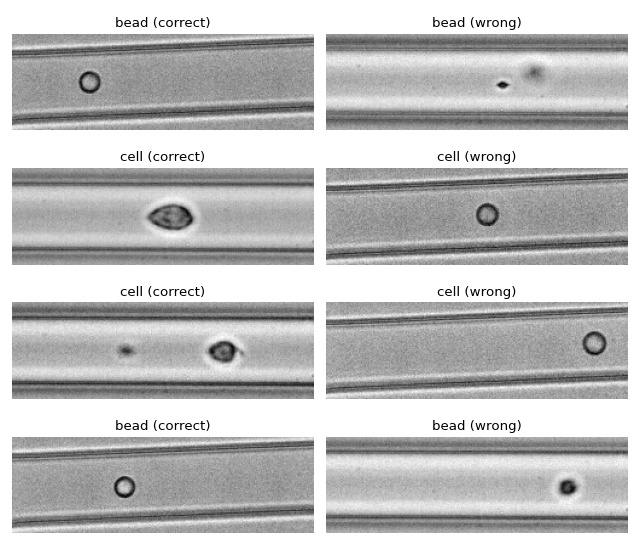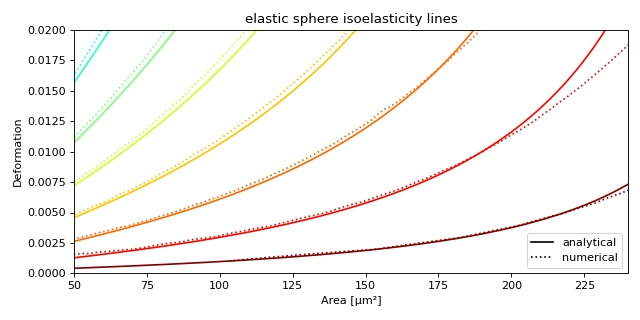# Examples¶

## Dataset overview plot¶

This example demonstrates basic data visualization with dclab and matplotlib. To run this script, download the reference dataset calibration_beads.rtdc [RHMG19] and place it in the same directory.

You will find more examples in the advanced usage section of this documentation.overview_plot.py

  1 2 3 4 5 6 7 8 9 10 11 12 13 14 15 16 17 18 19 20 21 22 23 24 25 26 27 28 29 30 31 32 33 34 35 36 37 38 39 40 41 42 43 44 45 46 47 48 49 50 51 52 53 54 55 56 57 58 59 60 61 62 63 64 65 66 67 68 69 70 71 72 73 74 75 76 77 import matplotlib.pylab as plt import numpy as np import dclab # Dataset to display DATASET_PATH = "calibration_beads.rtdc" # Features for scatter plot SCATTER_X = "area_um" SCATTER_Y = "deform" # Event index to display EVENT_INDEX = 100 xlabel = dclab.dfn.get_feature_label(SCATTER_X) ylabel = dclab.dfn.get_feature_label(SCATTER_Y) ds = dclab.new_dataset(DATASET_PATH) fig = plt.figure(figsize=(8, 7)) ax1 = plt.subplot(221, title="Simple scatter plot") ax1.plot(ds[SCATTER_X], ds[SCATTER_Y], "o", color="k", alpha=.2, ms=1) ax1.set_xlabel(xlabel) ax1.set_ylabel(ylabel) ax1.set_xlim(19, 40) ax1.set_ylim(0.005, 0.03) ax2 = plt.subplot(222, title="KDE scatter plot") sc = ax2.scatter(ds[SCATTER_X], ds[SCATTER_Y], c=ds.get_kde_scatter(xax=SCATTER_X, yax=SCATTER_Y, kde_type="multivariate"), s=3) plt.colorbar(sc, label="kernel density [a.u]", ax=ax2) ax2.set_xlabel(xlabel) ax2.set_ylabel(ylabel) ax2.set_xlim(19, 40) ax2.set_ylim(0.005, 0.03) ax3 = plt.subplot(425, title="Event image with contour") ax3.imshow(ds["image"][EVENT_INDEX], cmap="gray") ax3.plot(ds["contour"][EVENT_INDEX][:, 0], ds["contour"][EVENT_INDEX][:, 1], c="r") ax3.set_xlabel("Detector X [px]") ax3.set_ylabel("Detector Y [px]") ax4 = plt.subplot(427, title="Event mask with µm-scale") pxsize = ds.config["imaging"]["pixel size"] ax4.imshow(ds["mask"][EVENT_INDEX], extent=[0, ds["mask"].shape * pxsize, 0, ds["mask"].shape * pxsize], cmap="gray") ax4.set_xlabel("Detector X [µm]") ax4.set_ylabel("Detector Y [µm]") ax5 = plt.subplot(224, title="Fluorescence traces") flsamples = ds.config["fluorescence"]["samples per event"] flrate = ds.config["fluorescence"]["sample rate"] fltime = np.arange(flsamples) / flrate * 1e6 # here we plot "fl?_raw"; you may also plot "fl?_med" ax5.plot(fltime, ds["trace"]["fl1_raw"][EVENT_INDEX], c="#15BF00", label="fl1_raw") ax5.plot(fltime, ds["trace"]["fl2_raw"][EVENT_INDEX], c="#BF8A00", label="fl2_raw") ax5.plot(fltime, ds["trace"]["fl3_raw"][EVENT_INDEX], c="#BF0C00", label="fl3_raw") ax5.legend() ax5.set_xlim(ds["fl1_pos"][EVENT_INDEX] - 2*ds["fl1_width"][EVENT_INDEX], ds["fl1_pos"][EVENT_INDEX] + 2*ds["fl1_width"][EVENT_INDEX]) ax5.set_xlabel("Event time [µs]") ax5.set_ylabel("Fluorescence [a.u.]") plt.tight_layout() plt.show() 

## Young’s modulus computation from data on DCOR¶

This example reproduces the lower right subplot of figure 10 in [Her17]. It illustrates how the Young’s modulus of elastic beads can be retrieved correctly (independent of the flow rate, with correction for pixelation and shear-thinning) using the area-deformation look-up table implemented in dclab (right plot). For comparison, the flow-rate-dependent deformation is also shown (left plot).

The dataset is loaded directly from DCOR and thus an active internet connection is required for this example.emodulus_dcor.py

  1 2 3 4 5 6 7 8 9 10 11 12 13 14 15 16 17 18 19 20 21 22 23 24 25 26 27 28 29 30 31 32 33 34 35 36 37 38 39 40 41 42 43 44 45 46 47 48 49 50 51 52 53 54 55 56 57 58 59 60 61 62 63 64 65 66 67 68 69 70 71 72 73 74 75 76 77 78 79 80 81 import dclab import matplotlib.pylab as plt # The dataset is also available on figshare # (https://doi.org/10.6084/m9.figshare.12721436.v1), but we # are accessing it through the DCOR API, because we do not # have the time to download the entire dataset. The dataset # name is figshare-12721436-v1. These are the resource IDs: ds_loc = ["e4d59480-fa5b-c34e-0001-46a944afc8ea", "2cea205f-2d9d-26d0-b44c-0a11d5379152", "2cd67437-a145-82b3-d420-45390f977a90", ] ds_list = [] # list of opened datasets labels = [] # list of flow rate labels # load the data for loc in ds_loc: ds = dclab.new_dataset(loc) labels.append("{:.2f}".format(ds.config["setup"]["flow rate"])) # emodulus computation ds.config["calculation"]["emodulus medium"] = ds.config["setup"]["medium"] ds.config["calculation"]["emodulus model"] = "elastic sphere" ds.config["calculation"]["emodulus temperature"] = \ ds.config["setup"]["temperature"] # filtering ds.config["filtering"]["area_ratio min"] = 1.0 ds.config["filtering"]["area_ratio max"] = 1.1 ds.config["filtering"]["deform min"] = 0 ds.config["filtering"]["deform max"] = 0.035 # This option will remove "nan" events that appear in the "emodulus" # feature. If you are not working with DCOR, this might lead to a # longer computation time, because all available features are # computed locally. For data on DCOR, this computation already has # been done. ds.config["filtering"]["remove invalid events"] = True ds.apply_filter() # Create a hierarchy child for convenience reasons # (Otherwise we would have to do e.g. ds["deform"][ds.filter.all] # everytime we need to access a feature) ds_list.append(dclab.new_dataset(ds)) # plot fig = plt.figure(figsize=(8, 4)) # box plot for deformation ax1 = plt.subplot(121) ax1.set_ylabel(dclab.dfn.get_feature_label("deform")) data_deform = [di["deform"] for di in ds_list] # Uncomment this line if you are not filtering invalid events (above) # data_deform = [d[~np.isnan(d)] for d in data_deform] bplot1 = ax1.boxplot(data_deform, vert=True, patch_artist=True, labels=labels, ) # box plot for Young's modulus ax2 = plt.subplot(122) ax2.set_ylabel(dclab.dfn.get_feature_label("emodulus")) data_emodulus = [di["emodulus"] for di in ds_list] # Uncomment this line if you are not filtering invalid events (above) # data_emodulus = [d[~np.isnan(d)] for d in data_emodulus] bplot2 = ax2.boxplot(data_emodulus, vert=True, patch_artist=True, labels=labels, ) # colors colors = ["#0008A5", "#A5008D", "#A50100"] for bplot in (bplot1, bplot2): for patch, color in zip(bplot['boxes'], colors): patch.set_facecolor(color) # axes for ax in [ax1, ax2]: ax.grid() ax.set_xlabel("flow rate [µL/s]") plt.tight_layout() plt.show() 

## ML: Using RT-DC data with tensorflow¶

We use tensorflow to distinguish between beads and cells using scalar features only. The example data is taken from a reference dataset on DCOR. The classification accuracy using only the inputs area_ratio, area_um, bright_sd, and deform reaches values above 95%.

Warning

This example neglects a lot of important aspects of machine learning with RT-DC data (e.g. brightness normalization) and it is a very easy task (beads are smaller than cells). Thus, this example should only be considered as a technical guide on how tensorflow can be used with RT-DC data.

Note

What happens when you add "bright_avg" to the features list? Can you explain the result?

Apparently, debris in the cell dataset is classified as beads. We could have gotten around that by filtering the input data before inference. In addition, some beads get classified as cells as well. This is a result of the limited features used for training/inference. Under normal cirumstances, you would investigate other features in order to improve the model prediction.ml_tensorflow.py

  1 2 3 4 5 6 7 8 9 10 11 12 13 14 15 16 17 18 19 20 21 22 23 24 25 26 27 28 29 30 31 32 33 34 35 36 37 38 39 40 41 42 43 44 45 46 47 48 49 50 51 52 53 54 55 56 57 58 59 60 61 62 63 64 65 66 67 68 69 70 71 72 73 74 75 76 77 78 import matplotlib.pylab as plt import numpy as np import tensorflow as tf from dclab.ml import tf_dataset tf.random.set_seed(42) # for reproducibility # https://dcor.mpl.mpg.de/dataset/figshare-7771184-v2 dcor_ids = ["fb719fb2-bd9f-817a-7d70-f4002af916f0", "f7fa778f-6abd-1b53-ae5f-9ce12601d6f8"] labels = [0, 1] # 0: beads, 1: cells features = ["area_ratio", "area_um", "bright_sd", "deform"] # obtain train and test datasets train, test = tf_dataset.assemble_tf_dataset_scalars( dc_data=dcor_ids, # can also be list of paths or datasets labels=labels, feature_inputs=features, split=.8) # build the model model = tf.keras.Sequential( layers=[ tf.keras.layers.Input(shape=(len(features),)), tf.keras.layers.Dense(128, activation='relu'), tf.keras.layers.Dense(32), tf.keras.layers.Dropout(0.2), tf.keras.layers.Dense(2) ], name="scalar_features" ) # fit the model to the training data loss_fn = tf.keras.losses.SparseCategoricalCrossentropy(from_logits=True) model.compile(optimizer='adam', loss=loss_fn, metrics=['accuracy']) model.fit(train, epochs=5) # show accuracy using test data (loss: 0.1139 - accuracy: 0.9659) model.evaluate(test, verbose=2) # predict classes of the test data probability_model = tf.keras.Sequential([model, tf.keras.layers.Softmax()]) y_test = np.concatenate([y for x, y in test], axis=0) predict = np.argmax(probability_model.predict(test), axis=1) # take a few exemplary events from true and false classification false_cl = np.where(predict != y_test) true_cl = np.where(predict == y_test) num_events = min(4, min(len(true_cl), len(false_cl))) false_images = tf_dataset.get_dataset_event_feature( dc_data=dcor_ids, feature="image", tf_dataset_indices=false_cl[:num_events], split_index=1, split=.8) true_images = tf_dataset.get_dataset_event_feature( dc_data=dcor_ids, feature="image", tf_dataset_indices=true_cl[:num_events], split_index=1, split=.8) fig = plt.figure(figsize=(8, 7)) for ii in range(num_events): title_true = ("cell" if y_test[true_cl[[ii]]] else "bead") + " (correct)" title_false = ("cell" if predict[false_cl[ii]] else "bead") + " (wrong)" ax1 = plt.subplot(num_events, 2, 2*ii+1, title=title_true) ax2 = plt.subplot(num_events, 2, 2*(ii + 1), title=title_false) ax1.axis("off") ax2.axis("off") ax1.imshow(true_images[ii], cmap="gray") ax2.imshow(false_images[ii], cmap="gray") plt.tight_layout() plt.show() 

## ML: Creating built-in models for dclab¶

The tensorflow example already showcased a few convenience functions for machine learning implemented in dclab. In this example, we want to go even further and transform the predictions of an ML model into an ancillary feature (which is then globally available in dclab).

A few things are different from the other example:

• We rename model to bare_model to make a clear distinction between the actual ML model (from tensorflow) and the model wrapper (see Using models in dclab).
• We turn the two-class problem into a regression problem for one feature only. Consequently, the loss function changes to “binary crossentropy” and for some inexplicable reason we have to train for 20 epochs instead of the previously 5 to achieve convergence in accuracy.
• Finally, and this is the whole point of this example, we register the model as an ancillary feature and perform inference indirectly by simply accessing the ml_score_cel feature of the test dataset.

The plot shows the test fraction of the dataset. The x-axis is (arbitrarily) set to area. The y-axis shows the sigmoid (dclab automatically applies a sigmoid activation if it is not present in the final layer; see dclab.ml.models.TensorflowModel.predict()) of the model’s output logits.ml_builtin.py

  1 2 3 4 5 6 7 8 9 10 11 12 13 14 15 16 17 18 19 20 21 22 23 24 25 26 27 28 29 30 31 32 33 34 35 36 37 38 39 40 41 42 43 44 45 46 47 48 49 50 51 52 53 54 55 56 57 58 59 60 61 62 63 64 65 66 67 68 69 70 71 72 73 74 75 76 77 78 79 80 81 82 83 84 85 86 87 88 89 90 91 92 93 94 95 import matplotlib.pylab as plt import numpy as np import tensorflow as tf import dclab.ml tf.random.set_seed(42) # for reproducibility # https://dcor.mpl.mpg.de/dataset/figshare-7771184-v2 dcor_ids = ["fb719fb2-bd9f-817a-7d70-f4002af916f0", "f7fa778f-6abd-1b53-ae5f-9ce12601d6f8"] labels = [0, 1] # 0: beads, 1: cells features = ["area_ratio", "area_um", "bright_sd", "deform"] tf_kw = {"dc_data": dcor_ids, "split": .8, "shuffle": True, } # obtain train and test datasets train, test = dclab.ml.tf_dataset.assemble_tf_dataset_scalars( labels=labels, feature_inputs=features, **tf_kw) # build the model bare_model = tf.keras.Sequential( layers=[ tf.keras.layers.Input(shape=(len(features),)), tf.keras.layers.Dense(128), tf.keras.layers.Dense(32), tf.keras.layers.Dropout(0.3), tf.keras.layers.Dense(1) ], name="scalar_features" ) # fit the model to the training data # Note that we did not add a "sigmoid" activation function to the # final layer and are training with logits here. We also don't # have to manually add it in a later step, because dclab will # add it automatically (if it does not exist) before prediction. loss_fn = tf.keras.losses.BinaryCrossentropy(from_logits=True) bare_model.compile(optimizer='adam', loss=loss_fn, metrics=['accuracy']) bare_model.fit(train, epochs=20) # show accuracy using test data (loss: 0.0725 - accuracy: 0.9877) bare_model.evaluate(test, verbose=2) # register the ancillary feature "ml_score_cel" in dclab dc_model = dclab.ml.models.TensorflowModel( bare_model=bare_model, inputs=features, outputs=["ml_score_cel"], output_labels=["Probability of having a cell"], model_name="Distinguish between cells and beads", ) dc_model.register() # Now we are actually done already. The only thing left to do is to # visualize the prediction for the test-fraction of our dataset. # This involves a bit of data shuffling (obtaining the dataset indices # from the "index" feature (which starts at 1 and not 0) and creating # hierarchy children after applying the corresponding manual filters) # which is less complicated than it looks. # create dataset hierarchy children for bead and cell test data bead_train_indices = dclab.ml.tf_dataset.get_dataset_event_feature( feature="index", dc_data_indices=, split_index=0, **tf_kw) ds_bead = dclab.new_dataset(dcor_ids) ds_bead.filter.manual[np.array(bead_train_indices) - 1] = False ds_bead.apply_filter() ds_bead_test = dclab.new_dataset(ds_bead) # hierarchy child with test fraction cell_train_indices = dclab.ml.tf_dataset.get_dataset_event_feature( feature="index", dc_data_indices=, split_index=0, **tf_kw) ds_cell = dclab.new_dataset(dcor_ids) ds_cell.filter.manual[np.array(cell_train_indices) - 1] = False ds_cell.apply_filter() ds_cell_test = dclab.new_dataset(ds_cell) # hierarchy child with test fraction fig = plt.figure(figsize=(8, 7)) ax = plt.subplot(111) plt.plot(ds_bead_test["area_um"], ds_bead_test["ml_score_cel"], ".", ms=10, alpha=.5, label="test data: beads") plt.plot(ds_cell_test["area_um"], ds_cell_test["ml_score_cel"], ".", ms=10, alpha=.5, label="test data: cells") leg = plt.legend() for lh in leg.legendHandles: lh._legmarker.set_alpha(1) ax.set_xlabel(dclab.dfn.get_feature_label("area_um")) ax.set_ylabel(dclab.dfn.get_feature_label("ml_score_cel")) ax.set_xlim(0, 130) plt.tight_layout() plt.show() 

## Plotting isoelastics¶

This example illustrates how to plot dclab isoelastics by reproducing figure 3 (lower left) of [MMM+17].isoelastics.py

  1 2 3 4 5 6 7 8 9 10 11 12 13 14 15 16 17 18 19 20 21 22 23 24 25 26 27 28 29 30 31 32 33 34 35 36 37 38 import matplotlib.pylab as plt import matplotlib.lines as mlines from matplotlib import cm import numpy as np import dclab # parameters for isoelastics kwargs = {"col1": "area_um", # x-axis "col2": "deform", # y-axis "channel_width": 20, # [um] "flow_rate": 0.04, # [ul/s] "viscosity": 15, # [mPa s] "add_px_err": False # no pixelation error } isos = dclab.isoelastics.get_default() analy = isos.get(method="analytical", **kwargs) numer = isos.get(method="numerical", **kwargs) plt.figure(figsize=(8, 4)) ax = plt.subplot(111, title="elastic sphere isoelasticity lines") colors = [cm.get_cmap("jet")(x) for x in np.linspace(0, 1, len(analy))] for aa, nn, cc in zip(analy, numer, colors): ax.plot(aa[:, 0], aa[:, 1], color=cc) ax.plot(nn[:, 0], nn[:, 1], color=cc, ls=":") line = mlines.Line2D([], [], color='k', label='analytical') dotted = mlines.Line2D([], [], color='k', ls=":", label='numerical') ax.legend(handles=[line, dotted]) ax.set_xlim(50, 240) ax.set_ylim(0, 0.02) ax.set_xlabel(dclab.dfn.get_feature_label("area_um")) ax.set_ylabel(dclab.dfn.get_feature_label("deform")) plt.tight_layout() plt.show()